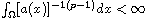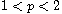Electron. J. Differential Equations, Vol. 2018 (2018), No. 190, pp. 1-18.

### Stability of weak solutions of a non-Newtonian polytropic filtration equation Huashui Zhan, Zhaosheng Feng

Abstract:
We study a non-Newtonian polytropic filtration equation with a convection term. We introduce new type of weak solutions and show the existence of weak solutions. We show that when, the stability of weak solutions is based on the usual initial-boundary value conditions. When, under the given conditions on the diffusion coefficient and the convection term, the stability of weak solutions can be proved without any boundary value condition. In particular, the stability results are presented based on the given optimal boundary value condition.

Submitted March 21, 2018. Published November 26, 2018.
Math Subject Classifications: 35K20, 35K65, 35R35.
Key Words: Weak solution; convection term; stability; boundary value condition.

Show me the PDF file (292 KB), TEX file for this article.Huashui Zhan School of Applied Mathematics Xiamen University of Technology Xiamen, Fujian 361024, China email: 2012111007@xmut.edu.cn Zhaosheng Feng Department of Mathematics University of Texas Rio Grande Valley Edinburg, TX 78539, USA email: zhaosheng.feng@utrgv.edu